# Python’s Modulo Operator

A short tutorial on things I learned last weekPhoto by Karim MANJRA on Unsplash

In Python, the modulo operator (signified as %) is one of seven arithmetic operators along with multiplication, division, addition, subtraction, exponentiation, and floor division. While reading through a blog on it, I learned some interesting facts about modulo that I’ll share here.

The basic application of the modulo is to represent the remainder after the division of one number — the numerator — by another number — the denominator.

`5 % 2 is 1Two goes into five two times with a remainder of 1.`

Just like regular math, there will be an error if 0 is the denominator.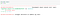Error if using 0

And modulo can be used with floats as well!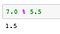Using modulo with floats

An alternative to the modulo operator is to use the math library: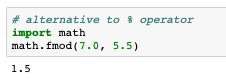Using the Math library

Interestingly, the math.fmod(x,y) is preferred over the modulo operator when working with floats. The precision is better.

Additionally, a negative float or integer can be used with the modulo operator or math.fmod(). The result takes the sign of the denominator, not the numerator. Key to keep in mind!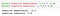Denominator determines the sign of the result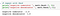True for fmod()

Python also has a built-in function called the divmod(a,b) that will return the quotient and remainder as a tuple when two, non-complex numbers are provided in the argument.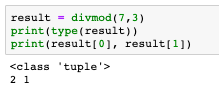Use divmod() to find quotient and remainder

Modulo is an excellent way to determine if a number is even or odd: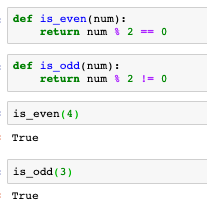Determine even or odd number

Finally, modulo can be used to evaluate code at specific intervals in conjunction with enumerate():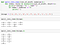Use of modulo